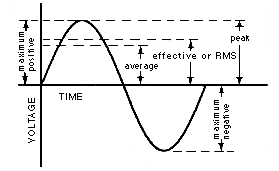ROOT MEAN SQUARE or ROOT-MEAN-SQUARE (RMS)

The square root of the average of the squares of a variable quantity.

In terms of voltage, the root mean square voltage is called the effective voltage, as opposed to the peak voltage which corresponds to the maximum AMPLITUDE of the voltage variations. RMS power (in watts) is similarly called the effective power, since for an AMPLIFIER, for instance, it represents its real POWER. See: SOUND PRESSURE.

The following relationships hold between peak, rms and average voltage. Note that the so-called peak-to-peak voltage is twice the peak voltage.

 rms voltage = 0.707 peak voltage rms voltage = 1.11 average voltage peak voltage = 1.414 rms voltage peak voltage = 1.57 average voltage average voltage = 0.637 peak voltage average voltage = 0.9 rms voltageRelative levels of average, RMS and peak voltages in a sine wave signal.

home